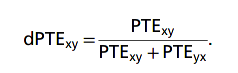Confusing values of normalized PTE (?)

Phase transfer entropy was introduced by Lobier et al., 2014, with this definition. PTE cannot be negative and its magnitude does not have a meaningful upper bound. To address this issue, a way to normalize PTE was introduced by Hillebrand et al., 2016. Where PTE values are normalized by the sum to the total (bidrectional) PTE between two signals.With this normalization, dPTE values range from 0 to 1, dPTExy + dPTEyx = 1, and the largest dPTE indicates the preferential flow of the information. This normalization is carried out in Brainstorm within the script PhaseTE_MF.

An additional step is carried on the dPTE values in the Brainstorm inside the script bst_connectivity, where 0.5 is subtracted from dPTE values to center them around 0. However, this centering seems problematic as it can lead to wrong interpretations:

For example, if dPTExy = 0.8 and dPTEyx = 0.2, this indicates a information goes preferentially from x to y. Removing 0.5 on both metrics results in dPTExy = 0.3 and dPTEyx = -0.3, while the numeric difference in the metrics is kept, the meaning does not look as clear. This is most notorious in the extreme case where dPTExy = 1 and dPTEyx = 0.

What is the reason of the zero-centering?
Is it a common practice?

Best,
Raymundo

Zero-centering of normalized PTE values has been removed from Brainstorm.